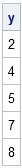In the R programming language, you can use a negative index in order to exclude an element from a list or a row from a matrix. For example, the syntax x[-1] means "all elements of x except for the first." In general, if v is a vector of indices to exclude, x[-v] contains the non-excluded elements.

This notation is not available in the SAS/IML language. However, you can use the SETDIF function to exclude elements easily. The SETDIF function computes the difference between two sets. (MATLAB also does not support negative indexes, but has a setdiff function. Notice that the MATLAB function is spelled with two F's, whereas the SAS/IML function has a single F.)

If a vector has N elements, then x[setdif(1:n, 1)] excludes the first element and x[setdif(1:n, v)] excludes all elements in the vector v. For example, the following syntax excludes the first, third, and sixth element of a vector:

```proc iml; v = {1 3 6}; /* exclude these elements */ x = T(1:8); y = x[setdif(1:8, v)]; print y;```You can use the SETDIF function in conjunction with the LOC function to exclude observations that satisfy some criterion:

```z = {., 2, ., 4, 5, ., 7, 8}; v = loc(z=.); /* find missing values, v = {1 3 6} */ y = z[setdif(1:ncol(z), v)]; /* keep non-missing values */```

You can also use the technique to exclude rows or columns of a matrix. For example, for each iteration of the following DO loop, B is matrix that is obtained from A by excluding the ith column:

```A = shape(1:30, 5); /* 5 x 6 matrix */ do i = 1 to ncol(A); cols = setdif(1:ncol(A), i); /* columns except i */ B = A[,cols]; /* exclude i_th column */ end;```
Share